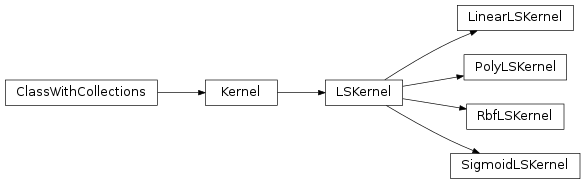# mvpa2.kernels.libsvm¶

PyMVPA LibSVM-based kernels

These kernels do not currently have the ability to run the calculations, so they are not translateable to other kernel types. They are implemented solely to standardize the interface between other kernel machines.Classes

 `LSKernel`(*args, **kwargs) A Kernel object which dictates how LibSVM will calculate the kernel `LinearLSKernel`(*args, **kwargs) A simple Linear kernel: K(a,b) = a*b.T `PolyLSKernel`(**kwargs) Polynomial kernel: K(a,b) = (gamma*a*b.T + coef0)**degree `RbfLSKernel`(**kwargs) Radial Basis Function kernel (aka Gaussian): `SigmoidLSKernel`(**kwargs) Sigmoid kernel: K(a,b) = tanh(gamma*a*b.T + coef0)